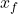## 4. An “extreme” pogo stick utilizes a spring whose uncompressed length is 46 cm and whose force constant is 1.4 x 104 N/m. A 60-kg pers

Question

4.
An “extreme” pogo stick utilizes a spring whose uncompressed length is 46 cm and whose force constant is 1.4 x 104 N/m. A 60-kg person is jumping on the pogo stick,
compressing the spring to a length of only 5.0 cm at the bottom of their jump. Which is the upward acceleration of the person at the moment the spring reaches its greatest
compression at the bottom of their jump?
6 m 2​

in progress 0
5 months 2021-08-13T22:26:49+00:00 1 Answers 4 views 0

## Answers ( )

a = 85.9 m / s²

Explanation:

For this exercise we can use Newton’s second law in the most compressed part

F – W = m a

force is the spring elastic force

F = – k Δx

k Δx – m g = m a

a = k/m  Δx – g

Δx = x₀ –ΔX = 46 – 5 = 41cm (1m / 100cm) = 0.41  m

let’s calculate

a = 1.4 10⁴/60 0.41 – 9.8

a = 85.9 m / s²